# Examples for 7th grade (seventh)

#### Number of examples found: 1974

• Perpendicular prism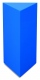Calculate the volume of the perpendicular prism if its height is 60.8 cm and the base is a rectangular triangle with 40.4 cm and 43 cm legs.
• Milk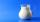At the kindergarten, every child got 1/5 liter of milk in the morning and another 1/8 liter of milk in the afternoon. How many liters were consumed per day for 20 children?
• Simple equation 2Find X in this simple equation: X/9 = 96/108
• RatiosReduce the numbers: 50 in a 1:2 ratio 111 at a ratio of 2:3 70 at 10:50 560 at a ratio of 3:8
• Number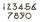What number I think? The third is 6 and half is 2 more than the its quarter.
• Cube 8The surface of the cube is 0.54 m2. Calculate the length of the cube edge.
• Series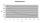Andrew wrote this series of numbers: 1,3,7,15, .... n. Which number in the series does not belong? ?
• Conference148 is the total number of employees. The conference was attended by 22 employees. How much is it in percent?
• Clock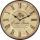What time is now, if the time elapsed after noon is 2/10 of time that elapses before midnight?
• Median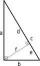In the right triangle are sides a=41 dm b=42 dm. Calculate the length of the medians tc to the hypotenuse.
• Carbon dioxide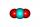Calculate how many grams of oxygen are in 50 g of carbon dioxide CO2. Relative atomic mass of oxygen is 16 and of carbon is 12.
• Ducats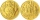The king divided the ducats to his three sons in a ratio of 2: 5: 4. How many ducats the king divided them if the youngest received 260 ducats, which was the least of all sons.
• Michal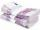Michal has 2.90 per hour. How much does he earn during the week of the summer job?
• Divide moneyDivide 1200 USD at a ratio of 1:2:3:4:5:6:9:10
• Water lakeThe length of the lake water is 8 meters width 7 meters and depth 120 centimeters. How many liters of water can fit into the water lake?
• Tablecloth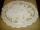I embroider tablecloth in 20 days but if I embroider 3/4 hours a day I have embroider it for 15 days. How long do I embroider a day?
• The largest numberFind the largest integer such that: 1. No figures is not repeat, 2. multiplication of every two digits is odd, 3. addition all digits is odd.
• Mother and daughter 2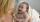The mother is 40 years older than her daughter. How old is the mother if her age is eight thirds age of daughter?
• Bases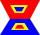The length of the bases trapezium are in ratio 4:5. Length of midline is 15. How long are the bases of a trapezoid?
• Two gears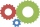The two gears fit together. The larger gear has 32 teeth, the smaller has 20 teeth less. How many times does turn a smaller gear if the bigger gear turns three times?

Do you have an interesting mathematical word problem that you can't solve it? Submit a math problem, and we can try to solve it.

We will send a solution to your e-mail address. Solved examples are also published here. Please enter the e-mail correctly and check whether you don't have a full mailbox.

Please do not submit problems from current active competitions such as Mathematical Olympiad, correspondence seminars etc...